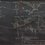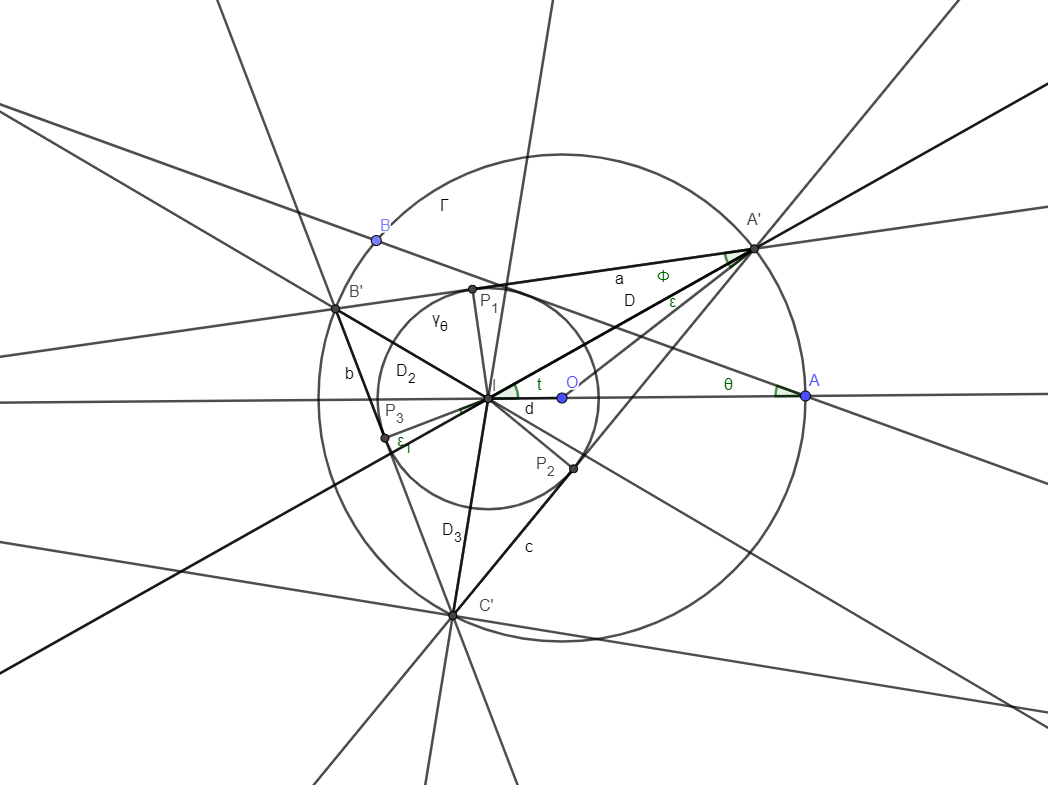# An extension of Poncelet's porism

A special case of Poncelet's porism states that whenever a triangle in inscribed in a circle and circumscribes another circle, there exist an infinite family of such triangles.

We can parametrise the possible inner circles with $\theta \in [0, \frac{\pi}{2})$ like so:

Let $\Gamma$ be the unit circle centred at $O = (0,0)$. Call the point $(1,0) A$. Pick a point $B$ on the upper half of $\Gamma$, such that $\angle OAB = \theta$. Reflect $B$ about the x-axis; call this point $C$. Let the incircle of $\triangle ABC$ be $\gamma_\theta$; its radius is $r = 2 \sin \theta + \cos 2 \theta - 1$, and it is centred at $(2 \sin \theta - 1, 0)$.

Find a parametrisation for the infinite family of triangles inscribed in $\Gamma$ and circumscribing $\gamma_\theta$. Then find the formula for the area of any given triangle, and determine when the area attains maxima/minima.Note by Jake Lai
1 year, 1 month ago

This discussion board is a place to discuss our Daily Challenges and the math and science related to those challenges. Explanations are more than just a solution — they should explain the steps and thinking strategies that you used to obtain the solution. Comments should further the discussion of math and science.

When posting on Brilliant:

• Use the emojis to react to an explanation, whether you're congratulating a job well done , or just really confused .
• Ask specific questions about the challenge or the steps in somebody's explanation. Well-posed questions can add a lot to the discussion, but posting "I don't understand!" doesn't help anyone.
• Try to contribute something new to the discussion, whether it is an extension, generalization or other idea related to the challenge.
• Stay on topic — we're all here to learn more about math and science, not to hear about your favorite get-rich-quick scheme or current world events.

MarkdownAppears as
*italics* or _italics_ italics
**bold** or __bold__ bold
- bulleted- list
• bulleted
• list
1. numbered2. list
1. numbered
2. list
Note: you must add a full line of space before and after lists for them to show up correctly
paragraph 1paragraph 2

paragraph 1

paragraph 2

[example link](https://brilliant.org)example link
> This is a quote
This is a quote
    # I indented these lines
# 4 spaces, and now they show
# up as a code block.

print "hello world"
# I indented these lines
# 4 spaces, and now they show
# up as a code block.

print "hello world"
MathAppears as
Remember to wrap math in $$ ... $$ or $ ... $ to ensure proper formatting.
2 \times 3 $2 \times 3$
2^{34} $2^{34}$
a_{i-1} $a_{i-1}$
\frac{2}{3} $\frac{2}{3}$
\sqrt{2} $\sqrt{2}$
\sum_{i=1}^3 $\sum_{i=1}^3$
\sin \theta $\sin \theta$
\boxed{123} $\boxed{123}$

## Comments

Sort by:

Top Newest

Thank you for this question. I found it fun to follow several different approaches. I only have a partial solution so far. I have used $d=2 \sin{θ}$ as the distance between the centres of $\mathbf{Γ}$ and $γ_{θ}$.Step 1. choose a parameterisation. I chose $t$ to be the angle between the x-axis and a line from $\mathbf{I}$, the centre of $γ_{θ}$ (and the incentre of $\mathbf{ABC}$) to an arbitrary point on $\mathbf{Γ}$ : $\mathbf{{A}'}$. The task is then to define a new triangle $\mathbf{{{A}'{B}'{C}'}}$ based on this parameter $t$.

Step 2. Calculate $D$, the distance from $\mathbf{I}$ to $\mathbf{Γ}$ along the line $\overline{\mathbf{I{A}'}}$.

$D = \sqrt{{d^{2} cos^{2} t - d^{2}+1}} - d \cos{t}$

Step 3. Calculate $a$, the distance from $\mathbf{{A}'}$ to $\mathbf{P_{1}}$ or $\mathbf{P_{2}}$, the tangent points of $\mathbf{{A}'}$ on $γ_{θ}$.

$a = \sqrt{{D^{2} - r^{2}}}$

Step 4. Calculate angle $\epsilon$, the angle between $\overline{\mathbf{O{A}'}}$ and $\overline{\mathbf{I{A}'}}$ where $\mathbf{O}$ is the centre of $\mathbf{Γ}$ and the circumcentre of $\mathbf{ABC}$.

$\epsilon = sin^{-1}(d sin{t})$

Step 5. calculate angle $\phi$, the angle between $\overline{\mathbf{I{A}'}}$ and $\overline{\mathbf{P_{1}{A}'}}$.

$\phi = sin^{-1}(\frac{D}{r})$

Step 6. Note that $\mathbf{P_{3}}$, the third point of tangency between $\mathbf{{{A}'{B}'{C}'}}$ and $γ_{θ}$ lies at an angle $t + π - \epsilon$ from $\mathbf{I}$. The angles $t_{2}$ and $t_{3}$ from $\mathbf{I}$ to $\mathbf{{B}'}$ and $\mathbf{{C}'}$ respectively bisect the angles $\mathbf{P_{1}IP_{3}}$ and $\mathbf{P_{2}IP_{3}}$ respectively so:

$t_{2} = t + \frac{3\pi}{4} - \frac{\phi}{2} - \frac{\epsilon}{2}$

$t_{3} = t + \frac{\pi}{4} + \frac{\phi}{2} - \frac{\epsilon}{2}$

Step 7. Calculate $D_{2}$ and $D_{3}$, the distances from $\mathbf{I}$ to $\mathbf{{B}'}$ and $\mathbf{{C}'}$ respectively by the formula in step 2 (using $t_{2}$ and $t_{3}$ in place of $t$) and then calculate $b$ and $c$ using the same method as the calculation of $a$.

Step 8. We now have a description of $\mathbf{{{A}'{B}'{C}'}}$. The area is $r(a+b+c)$ and the perimeter is $2(a+b+c)$. Area and perimeter are maximised when the "$-d cos{t}$" terms in steps 2 and 7 are maximised. If $d$ is positive, this happens when $t=\pi$ and if $d$ is negative when $t=0$. So for small $θ$, triangle $\mathbf{ABC}$ is of larger area than any other $\mathbf{{{A}'{B}'{C}'}}$. For large $θ$, triangle $\mathbf{ABC}$ is smaller than any other $\mathbf{{{A}'{B}'{C}'}}$ with equality at $\theta=\frac{\pi}{6}$ where $\mathbf{ABC}$ is an equilateral triangle and every $\mathbf{{{A}'{B}'{C}'}}$ is of equal area and all have the greatest area of any triangle at any $θ$.

- 1 year ago

Log in to reply

×

Problem Loading...

Note Loading...

Set Loading...